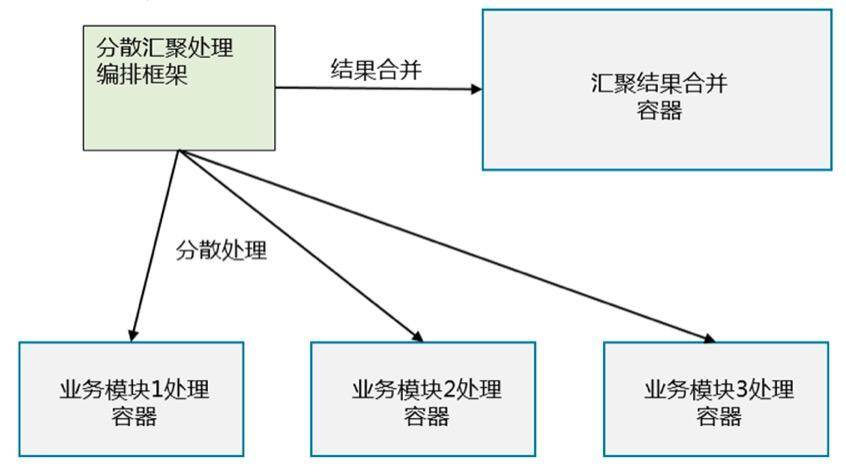# K8s 容器设计模式实践，分散收集模式

《Kubernetes 与云原生应用》专栏是 InfoQ 向轻元科技首席架构师王昕约稿的系列文章。本专栏包含 8 篇内容，将会从介绍和分析 Kubernetes 系统以及云原生应用入手，逐步推出基于 Kubernetes 的容器设计模式实践案例，希望对计划应用 Kubernetes 的朋友有所帮助。本文是该专栏的第 7 篇。

1. Kubernetes 系统架构与设计理念
1. 云原生应用的设计理念与挑战
1. Kubernetes 与云原生应用的容器设计模式
1. Kubernetes 容器设计模式实践案例 - 单节点多容器模式
1. Kubernetes 容器设计模式实践案例 - 多节点选举模式
1. Kubernetes 容器设计模式实践案例 - 工作队列模式
1. Kubernetes 容器设计模式实践案例 - 分散收集模式
1. 云原生应用的容器设计模式综述与展望

## 分散收集模式Fig01 分散收集模式示意图

## 分布式计算模式项目 Metapaticle

Metapaticle 项目的目的在开发并展示一种基础设施即代码的编程模式，力图将基础设施管理逻辑和功能运算逻辑以同一种语言实现在同一短小精干的程序中。

Metaparticle 支持 3 种分布式系统的服务模式：分散收集模式（Scatter/Gather）、分片模式（Shard）和水平扩展模式（Spread）。其中分散收集模式是更具通用性的一种模式，正好也是本系列文章中准备介绍的最后一种容器设计模式，也是最复杂的一种设计模式。本文中针对分散收集模式的演示案例，以 metaparticle 为基础，先要通过 nodejs 的包管理工具安装 metaparticle 模块，然后通过 node 来试验本文中的案例。

## 安装 Metaparticle

$npm install metaparticle 也可以下载代码后，通过本地代码安装： $ git clone  https://github.com/xwangqingyuan/metaparticle.git
$npm install ./metaparticle/ 在安装过程中如果碰到缺少的nodejs module，直接用npm install即可。 ## 通过分散收集计算正态分布（高斯分布）的统计特性 在本演示案例中，利用分散收集模式模拟展示一个正态分布的统计直方图。 计算高斯分布的示例代码文件 scatter-gather-gaussian.js 清单。 // Import the main library var mp = require('metaparticle'); // A simple function for calculating a Gaussian distributed value // from a uniform random value var gaussian = function (sigma, mean) { var u1 = 2 * Math.PI * Math.random(); var u2 = -2 * Math.log(Math.random()); var n = Math.sqrt(u2) * Math.cos(u1); return n * sigma + mean; }; // This function is executed on each leaf var leafFunction = function (data) { var result = { 'n': [] }; for (var i = 0; i < 100; i++) { result.n.push(gaussian(25, 100)); } return result; }; // This function is executed on each root var mergeFunction = function (responses) { var histogram = [0, 0, 0, 0, 0, 0, 0, 0, 0, 0]; for (var i = 0; i < responses.length; i++) { for (var j = 0; j < responses[i].n.length; j++) { if (responses[i].n < 0 || responses[i].n > 200) { continue } var ix = Math.floor(responses[i].n[j] / 20); histogram[ix]++; } } return histogram; }; var svc = mp.service( // name of the service "histogram-service", // library function that creates a scatter/gather service mp.scatter(10, leafFunction, mergeFunction)); // Expose the root service to the world svc.subservices.gather.expose = true; // And serve mp.serve(); 在本例中，根服务器将计算任务分配给 10 个叶子服务器计算。方法mp.scatter用来调度整体的分布式计算。方法mp.scatter的第一个参数是分片的个数，第 2 个参数是叶子服务器的计算函数leafFunction，第 3 个参数是根服务器合并的计算函数mergeFunction 在本例中，每个叶子节点都模拟一个方差为 25，均值为 100 的正态分布随机变量。计算函数leafFunction用来产生 100 个随机变量，计算函数mergeFunction用来合并所有子节点的随机变量，统计这些随机变量的样本值处在不同区间的个数。 启动分散收集模式计算高斯分布的服务器端容器服务。 xwang@dev-xwang:/gitws/github.com/xwangqingyuan/metaparticle/examples$
node scatter-gather-gaussian.js
building image (this may take a bit)
building image done.
deploying

xwang@dev-xwang:/gitws/github.com/xwangqingyuan/metaparticle/examples$node client.js histogram-service [0,4,55,139,296,284,160,53,9,0] 通过计算结果可以看到，正如一般正态分布所预期的一样，正态分布的主要样本个数集中在直方图中间的部分296 和 284，越向两边样本个数越少。 停止并删除分散收集模式的服务器端容器服务。 xwang@dev-xwang:/gitws/github.com/xwangqingyuan/metaparticle/examples$
node scatter-gather-gaussian.js delete

## 通过分散收集计算均匀分布的统计特性

// Import the main library
var mp = require('metaparticle');
// A simple function to caculate a uniform integer
var uniform = function (lower, upper) {
return Math.round(lower + (upper-lower)*Math.random());
};
// This function is executed on each leaf
var leafFunction = function (data) {
var result = { 'n': [] };
for (var i = 0; i < 100; i++) {
result.n.push(uniform(0, 200));
}
return result;
};
// This function is executed on each root
var mergeFunction = function (responses) {
var histogram = [0, 0, 0, 0, 0, 0, 0, 0, 0, 0];
for (var i = 0; i < responses.length; i++) {
for (var j = 0; j < 10; j++) {
histogram[j] += responses[i].n[j];
}
}
return histogram;
};
var svc = mp.service(
// name of the service
"histogram-service",
// library function that creates a scatter/gather service
mp.scatter(10, leafFunction, mergeFunction));
// Expose the root service to the world
svc.subservices.gather.expose = true;
// And serve
mp.serve();

xwang@dev-xwang:/gitws/github.com/xwangqingyuan/metaparticle/examples$node scatter-gather-uniform.js.js building image (this may take a bit) building image done. deploying 运行客户端程序发送请求计算统计结果。 xwang@dev-xwang:/gitws/github.com/xwangqingyuan/metaparticle/examples$
node client.js histogram-service
[1244,980,1066,961,679,1097,825,1021,719,911]

xwang@dev-xwang:/gitws/github.com/xwangqingyuan/metaparticle/examples$node scatter-gather-uniform.js delete ## 通过分散收集计算整数中的数字出现频率 在本例中，利用分散收集模式，模拟统计一系列整数的 10 进制占位数字的统计特性。 在本例中，每个叶子节点都计算 100 个处于 0 到 100 之间的均匀分布的整数的所有占位数字的出现次数，例如 56 这个整数，有 5 和 6 两个数字，会给 5 和 6 这两个数字的次数分别增加 1。计算函数leafFunction用来产生 100 个随机变量，并统计这 100 个随机变量的数字（0-9）出现次数，计算函数mergeFunction用来合并所有子节点的计算的出现次数，计算出总的出现次数。 计算整数中的数字出现频率的示例代码文件 scatter-gather-count-digit.js 清单。 // Import the main library var mp = require('metaparticle'); // A simple function to caculate a uniform integer var uniform = function (lower, upper) { return Math.round(lower + (upper-lower)*Math.random()); }; // This function is executed on each leaf var leafFunction = function (data) { var result = {'n': [0, 0, 0, 0, 0, 0, 0, 0, 0, 0]}; for (var i = 0; i < 100; i++) { var num = uniform(0, 100); var str = "" + num; for (var j = 0; j < str.length; j++) { var digit = parseInt(str.charAt(j)); result.n[digit]++; } } return result; }; // This function is executed on each root var mergeFunction = function (responses) { var histogram = [0, 0, 0, 0, 0, 0, 0, 0, 0, 0]; for (var i = 0; i < responses.length; i++) { for (var j = 0; j < 10; j++) { histogram[j] += responses[i].n[j]; } } return histogram; }; var svc = mp.service( // name of the service "histogram-service", // library function that creates a scatter/gather service mp.scatter(10, leafFunction, mergeFunction)); // Expose the root service to the world svc.subservices.gather.expose = true; // And serve mp.serve(); 启动分散收集模式计算高斯分布的服务器端容器服务。 xwang@dev-xwang:/gitws/github.com/xwangqingyuan/metaparticle/examples$
node scatter-gather-count-digit.js
building image (this may take a bit)
building image done.
deploying

xwang@dev-xwang:/gitws/github.com/xwangqingyuan/metaparticle/examples$node client.js histogram-service [83,184,178,196,207,202,204,231,205,196] 通过计算结果可以看到，在所有数字中，1-9 的数字出现频率是比较接近的，大概在 200 左右，而 0 作为数字出现频率是较低的，大概少 100 个左右，这是因为 0 不会作为首位数字出现。 停止并删除分散收集模式的服务器端容器服务。 xwang@dev-xwang:/gitws/github.com/xwangqingyuan/metaparticle/examples$
node scatter-gather-count-digit.js delete

## 通过分散收集计算整数末尾数字的出现频率

// Import the main library
var mp = require('metaparticle');
// A simple function to caculate a uniform integer
var uniform = function (lower, upper) {
return Math.round(lower + (upper-lower)*Math.random());
};
// This function is executed on each leaf
var leafFunction = function (data) {
var result = {'n': [0, 0, 0, 0, 0, 0, 0, 0, 0, 0]};
for (var i = 0; i < 100; i++) {
var num = uniform(0, 20)*5;
var str = "" + num;
var digit = parseInt(str.charAt(str.length-1));
result.n[digit]++;
}
return result;
};
// This function is executed on each root
var mergeFunction = function (responses) {
var histogram = [0, 0, 0, 0, 0, 0, 0, 0, 0, 0];
for (var i = 0; i < responses.length; i++) {
for (var j = 0; j < 10; j++) {
histogram[j] += responses[i].n[j];
}
}
return histogram;
};
var svc = mp.service(
// name of the service
"histogram-service",
// library function that creates a scatter/gather service
mp.scatter(10, leafFunction, mergeFunction));
// Expose the root service to the world
svc.subservices.gather.expose = true;
// And serve
mp.serve();

xwang@dev-xwang:/gitws/github.com/xwangqingyuan/metaparticle/examples$node scatter-gather-count-last-digit.js building image (this may take a bit) building image done. Deploying 运行客户端程序发送请求计算统计结果。  xwang@dev-xwang:/gitws/github.com/xwangqingyuan/metaparticle/examples$
node client.js histogram-service [495,0,0,0,0,505,0,0,0,0]

xwang@dev-xwang:/gitws/github.com/xwangqingyuan/metaparticle/examples\$
node scatter-gather-count-last-digit.js delete

## 评论2018 年 12 月 13 日 15:04